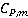### Create an Account

Already have account?

### Forgot Your Password ?

Home / Questions / Calculate ∆S if 100 mol of liquid water is heated from 000° to 100°C under constant pressure and if

# Calculate ∆S if 100 mol of liquid water is heated from 000° to 100°C under constant pressure and if

Calculate ∆S if 1.00 mol of liquid water is heated from 0.00° to 10.0°C under constant pressure and if= 75.3 J K–1 mol–1.

b. The melting point of water at the pressure of interest is 0.00°C and the enthalpy of fusion is 6.010 kJ mol–1. The boiling point is 100.°C and the enthalpy of vaporization is 40.65 kJ mol–1. Calculate ∆S for the transformation H2O(s, 0°C) H → 2O(g, 100.°C).

Jun 09 2020 View more View Less

#### Answer (Solved)Subscribe To Get Solution